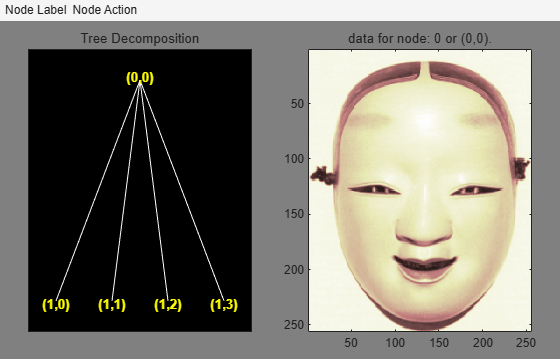# wpcoef

Wavelet packet coefficients

## Syntax

``x = wpcoef(wpt,n)``
``x = wpcoef(wpt)``

## Description

`wpcoef` is a one- or two-dimensional wavelet packet analysis function.

example

````x = wpcoef(wpt,n)` returns the coefficients associated with the node `n` of the wavelet packet tree `wpt`. If node `n` does not exist, `x = []`.```
````x = wpcoef(wpt)` is equivalent to `x = wpcoef(wpt,0)`.```

## Examples

collapse all

Load a 1-D signal. Save the current extension mode.

```load noisdopp x = noisdopp; origMode = dwtmode('status','nodisp');```

Use `dwtmode` to change the extension mode to zero-padding. Obtain the wavelet packet tree object corresponding to the 3-level wavelet packet decomposition of the signal using the `db1` wavelet. Plot the tree.

```dwtmode('zpd','nodisp') wpt = wpdec(x,3,"db1"); plot(wpt)```Obtain the coefficients at the node (3,0). Plot the signal and coefficients.

```cfs = wpcoef(wpt,[3 0]); subplot(2,1,1) plot(x) title('Signal') axis tight subplot(2,1,2) plot(cfs) title('Packet (3,0) Coefficients') axis tight```Load an image. Obtain the wavelet packet tree that corresponds to a one-level wavelet packet decomposition of the image using the `sym4` wavelet.

```load woman2 t = wpdec2(X,1,'sym4');```

Plot the tree.

`plot(t)`Obtain the coefficients at the node (1,0). Plot the coefficients.

```cfs = wpcoef(t,[1 0]); figure imagesc(cfs) title('Packet (1,0) Coefficients') colormap(pink)```Restore the extension mode to the original setting.

`dwtmode(origMode,'nodisp')`

## Input Arguments

collapse all

Wavelet packet tree, specified as a `wptree` object.

Node in a wavelet packet tree, specified as a nonnegative integer, or pair of nonnegative integers. See `depo2ind` and `ind2depo`.

Example: If `wpt = wpdec(1:256,2,"sym4")`, then `wpcoef(wpt,3)` and ```wpcoef(wpt,[2 0])``` specify the same node.

Data Types: `double`

## Output Arguments

collapse all

Node coefficients, returned as a vector or matrix.

Data Types: `double`